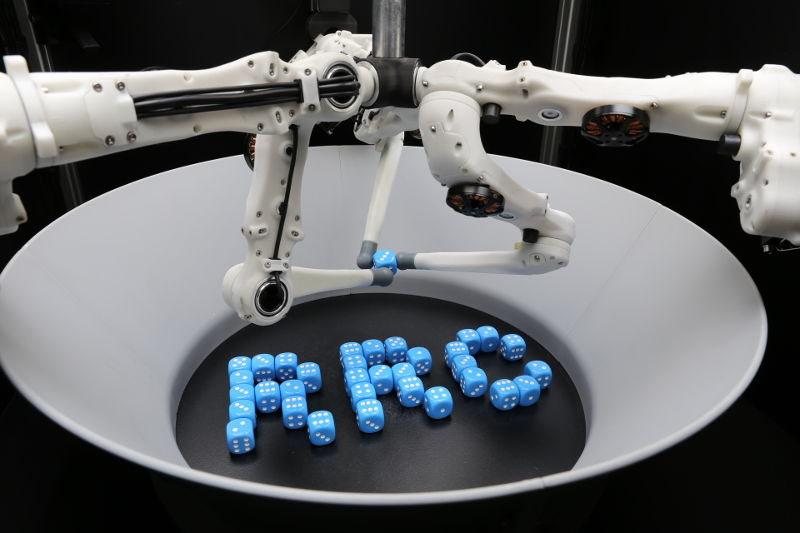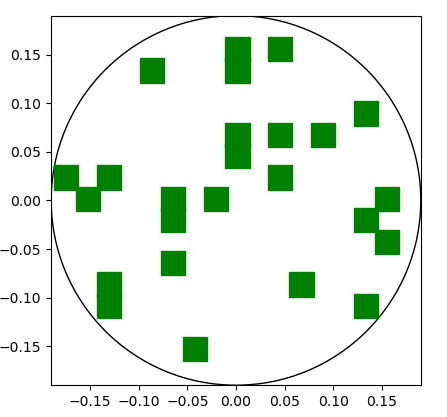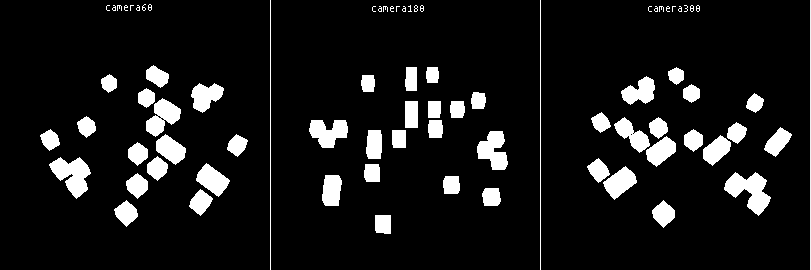The goal of this task is to arrange multiple dice into a given pattern.

The pattern is given as a list of N target positions where N is the number of dice:

goal = [
(0.10, 0.04, 0.01),
(0.04, -0.08, 0.01),
(0.0, 0.15, 0.01),
...
]

Since the single dice are indistinguishable the target positions are not linked to a specific die, there should just be one die at each position in the end.

The duration of a run is 120000 steps (~2 minutes). This value is also given by EPISODE_LENGTH.

The cost of each step is computed using the camera images. Based on the colour, it is determined how many “die pixels” are outside of the target regions (see evaluate_state()).

Duration of the episode in time steps (corresponds to ~2 minutes).

Number of dice in the arena

Sample a random list of die goal positions.

Compute cost of a given state. Less is better.

The cost is computed as the number of “die pixels” in the actual masks that do not overlap with the goal mask:

Parameters
• goal_masks (Sequence[numpy.ndarray]) – Masks of the desired die positions in the camera images, one mask per camera. Use generate_goal_mask() to generate the goal mask for a given goal.

• actual_masks (Sequence[numpy.ndarray]) – Masks of the actual die positions in the camera images, one mask per camera using the same order as goal_masks.

Returns

The cost of the given state.

Return type

float

Verify that the goal has the proper shape and all positions are valid.

Raises
• OutOfArenaError – If a die position is outside the valid range.

• InvalidGoalError – If the goal does not have the expected shape.

Load or sample a goal based on the given goal config file.

Parameters

filename (str) – Path to the goal config JSON file. If it contains an entry “goal”, its value is used as goal. Otherwise a random goal is sampled.

Returns

The goal as JSON-encoded string.

Return type

str

Set random seed for this module.

Parameters

seed (int) –

Visualise the target positions in 2d.

Shows a top-down view of the arena with the goal positions marked by squares.

Parameters

target_positions (Sequence[Sequence[float]]) – The goal that is visualised.

Example on how the visualisation look like:Generate goal masks that can be used with evaluate_state().

A goal mask is a single-channel image where the areas at which dice are supposed to be placed are white and everything else is black. So it corresponds more or less to a segmentation mask where all dice are at the goal positions.

For rendering the mask, TARGET_WIDTH is used for the die width to add some tolerance.

Parameters
• camera_parameters (Sequence[trifinger_simulation.camera.CameraParameters]) – List of camera parameters, one per camera.

• goal (Sequence[Sequence[float]]) – The goal die positions.

Returns

List of masks. The number and order of masks corresponds to the input camera_parameters.

Return type

List[numpy.ndarray]

Example on how the generated goal masks look like: